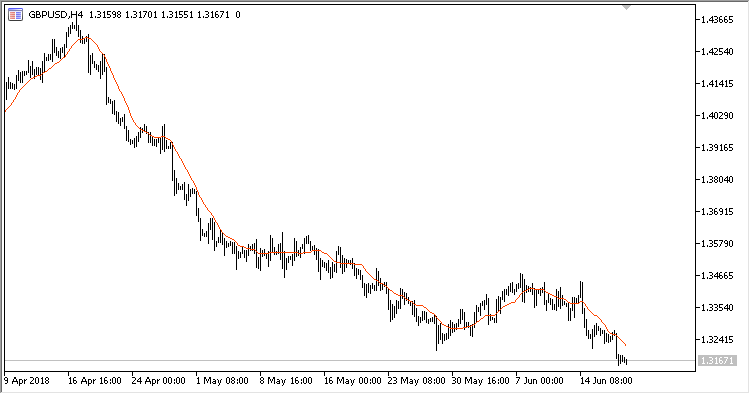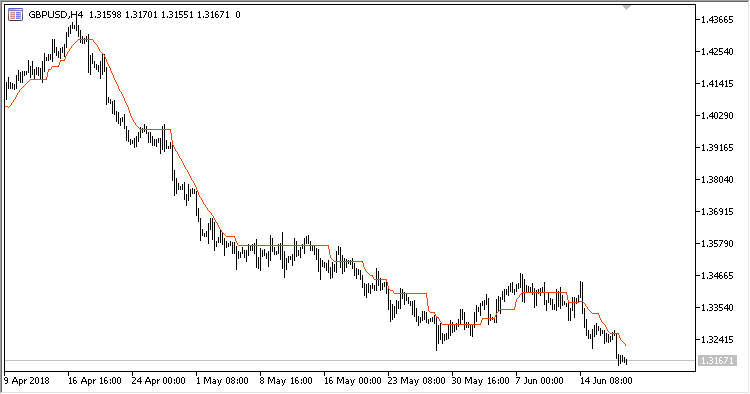# XMA – indicator for MetaTrader 5

• A+
category：MT5 INDICATORS

Indicator XMA (Digital adaptive Moving Average) is a digital adaptive moving average XMA. It calculates the moving aberage using a digital filter.

It only changes the value of the moving average from bar to bar, if the new value is the step size (in points) higher than the previous one.

It has four configurable parameters:

• Period - calculation period;
• Price X step - price step in points;
• Method - calculation method;
• Applied price - price used for calculations.

Calculations:

If Abs(MA-PrevMA) >= Price X step:

`XMA = MA`

Otherwise:

`XMA = PrevXMA`

where:

`MA - MA(Applied price, Period, Method)`Fig.1 Price X step = 20Fig.2 Price X step = 60